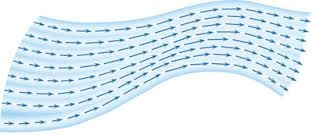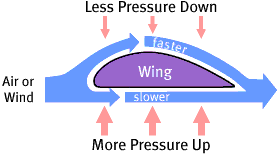# Mechanical properties of fluids and Bernoulli's principle in brief

3 years ago

A flowinmg liquid may be regarded as consisting of a number of layers one above the other.Fluid Mechanics is of fundamental importance as it talks both about the fluids both at rest and in motion.### Steady Flow (Stream Line Flow)

It is the flow in which the velocity of fluid particles crossing a particular point is the same at all the times. Thus, each particle takes the same path as taken by a previous particle through the point.

### Steady, Streamline or Laminar FlowThe flow of fluid is said to be streamline if the velocity at any point in the fluid remains constant with time (in magnitude as well as in direction) in this case energy needed to drive the fluid is used up in overcoming the viscous force between its layers. All particles passing through a point in a steady flow follow the same path. The paths are known as streamlines> An example is water slowly flowing through a pipe.

### Turbulent Flow

When the motion of a particle varies rapidly in magnitude and direction, the flow is said to be turbulent. In other words, when the velocity exceeds beyond the critical velocity, the paths and velocities of liquid change continuously and haphazardly. This flow is called turbulent flow. An example is water coming out of a fountain.

### Critical Velocity

If in the case of a steady flow of fluids the velocity of flow is gradually increased it is found that the motion remains steady (streamline or laminar) upto a certain limit. If the velocity of flow crosses a certain limit the fluid particles do not follow the path of the preceding one and the motion becomes turbulent. The maximum velocity upto which fluid motion remains steady is called critical velocity. According to Reynold, in case of motion of fluids in narrow tubes, critical velocity depends on the density r and coefficient of viscosity η of the fluid as well as radius of the tube. i.e.

Vc  = η/rρ     or   Vc = R η/ρr

Here R is a dimensionless constant called Reynold's number. For steady flow R 3000 flow is turbulent.

## Bernoulli's Theorem

###“Bernoulli's principle thus says that a rise (fall) in pressure in a flowing fluid must always be accompanied by a decrease (increase) in the speed, and conversely, if an increase (decrease) in , the speed of the fluid results in a decrease (increase) in the pressure”.

Consider a tube of flow, ABCD. In a time Δt, liquid moves and the liquid element becomes A'B'C'D'. In other words, we can also interpret that ABB'A' has gone to DCC'D'.Δm = ρA1v1 Δt = ρ A2v2 Δt

Work done by fluid pressure at end 1 = (P1A1)v1 Δt = P1 Δm/r

Work done by fluid pressure at end 2 = -(P2A2)v2 Δt = -P2 Δm/r

Work done by gravity = -(Δm)g(h2 - h1)

Change in kinetic energy = (1/2) Δm [v22 - v12]

Using, work-energy theorem (W = ΔK),P1 Δm/ρ - P2 Δm/ρ - Δmg (h2 - h1) = 1/2 Δm [V22 - V12]

Or  p1/ρ + gh+ V12/2 = P2 + gh2 + V22/2

Or  P1 + ρgh1 + ρV12/2 = P2 + ρgh2 + ρV22/2

Or,In a streamline flow of an ideal fluid, the sum of pressure energy per unit volume, potential energy per unit volume and kinetic energy per unit volume is always constant at all cross sections of the liquid.

Bernoulli's equation is valid only for incompressible steady flow of a fluid with no viscosity.